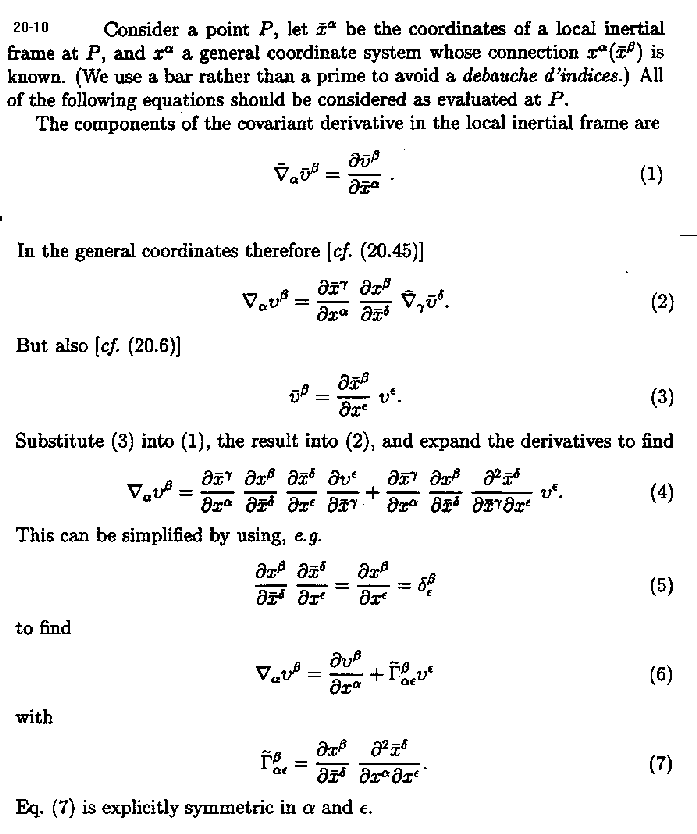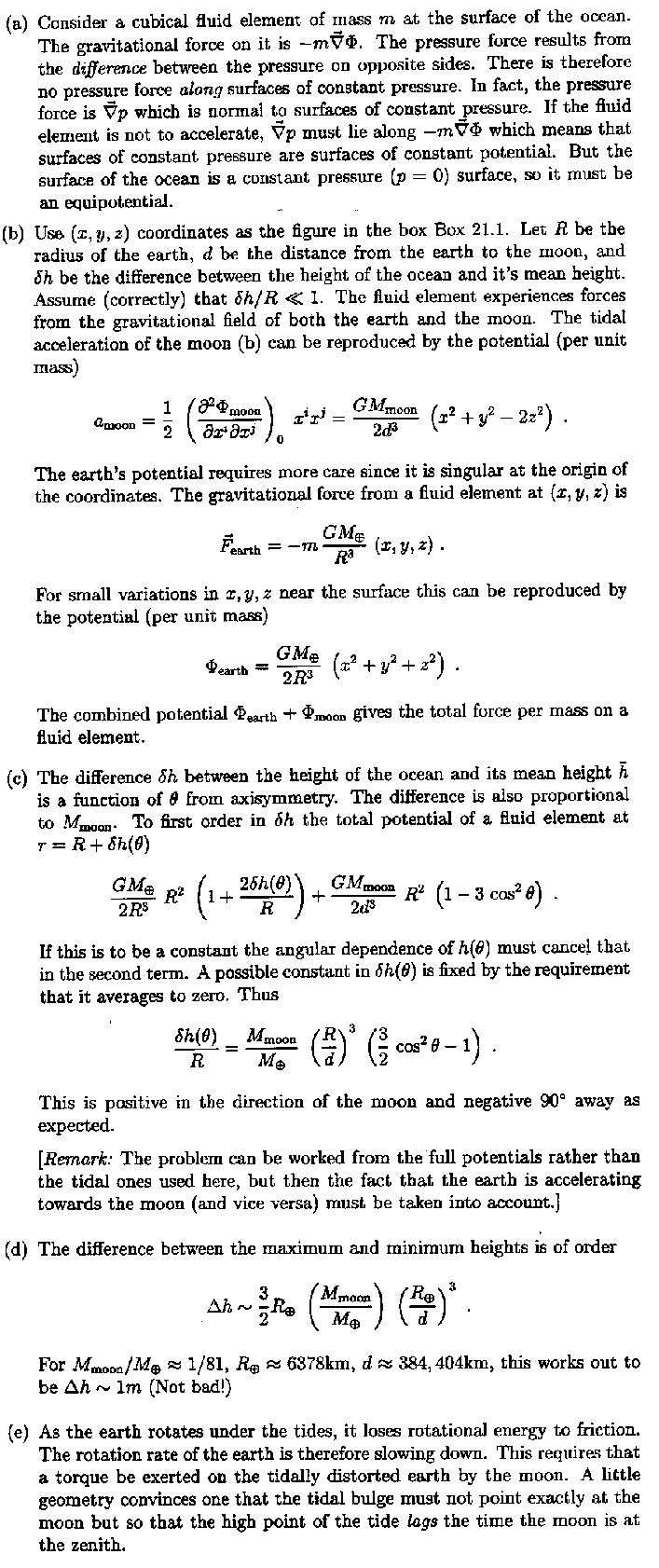20-18 The covariant components are

xa = gab xb = ga1
Substitute this into the formula (20.68) for the covariant derivative to find
 čb xa = 1 2 µĶ Čga1 Čxb - Čgb1 Čxa + Čgab Čx1 ÷° .
The first term is antisymmetric in (a, b), and the last term vanishes because of x1-independence. Thus the symmetrized čb xa vanishes - that's Killing's equation. (This does not, of course, prove that every vectorfield generating a symmetry of the metric satisfies Killing's equation - but it is so :)

20-20
a) In rectangular coordinates the Christoffel symbols vanish, so Killing's equation becomes xa,b + xb,a = 0. The first two have constant components, whose derivatives vanish, for the third, for example, the (1, 2) component is (-y),y + x,x = -1 + 1 = 0.

b) If you verify this by starting with Č/Čf, you will be doing problem 8-8 over again; else, similarly but "the other way round", express Č/Čx in terms of Č/Čr and Č/Čf.

c) Let the symmetry origin be at (X, Y), so coordinates centered there are xó = x - X,     yó = y - Y. The desired K. V. is
 hó = - yó Č Čxó + xó Č Čyó = h - X Č Čy + Y Č Čx .

21-221-11 Hartle's official answer is: "Running the Mathematica program Curvature and the Einstein Equations for the metric given in Problem 8.12 gives R = -2."